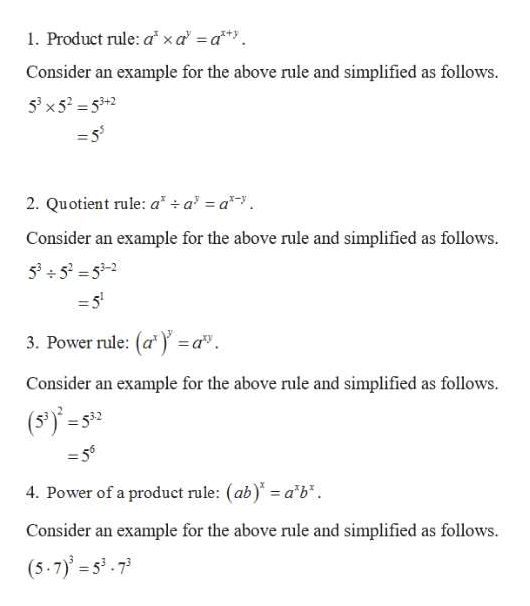# i need help with simplifying exponets

Question

i need help with simplifying exponets

check_circleExpert Solution
Step 1

Exponents rules with examples ar...help_outlineImage Transcriptionclose1. Product rule: a x a a Consider an example for the above rule and simplified as follows. 5x5-53+2 2. Quotient rule: a +a a Consider an example for the above rule and simplified as follows. 5353-2 3. Power rule: (a= a Consider an example for the above rule and simplified as follows. (S)-s3 -56 4. Power of a product rule: (ab) a'b Consider an example for the above rule and simplified as follows. (5.7)-573 fullscreen

### Want to see the full answer?

See Solution

#### Want to see this answer and more?

Solutions are written by subject experts who are available 24/7. Questions are typically answered within 1 hour*

See Solution
*Response times may vary by subject and question
Tagged in

### Algebra